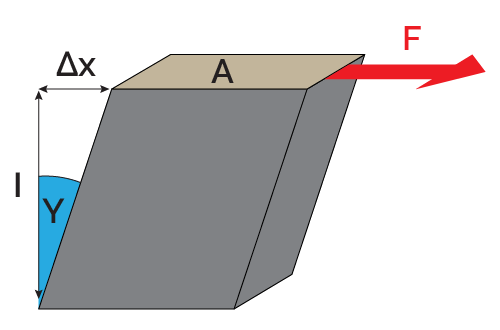# Shear Modulus Calculator

Created by Luis Fernando
Reviewed by Steven Wooding
Last updated: May 24, 2022

Welcome to our shear modulus calculator, where you'll be able to calculate the shear modulus of a cubic element subjected to a force tangent to its area.

The shear modulus, also known as the modulus of rigidity, is a material property used in many applications. For example:

• In our shear strain calculator, the shear modulus is necessary to find the shear strain due to shear stress.
• It's also needed to calculate the twist angle in shafts subjected to torsion.
• From the theoretical point of view, it has great relevance, as shear modulus, Young's modulus, and Poisson's ratio are the constants that form the generalized Hook's law for homogenous isotropic materials.

Read on to know the modulus of rigidity equation used by this calculator and the modulus of rigidity of steel and many other materials.

## Shear modulus formula

Although an idealization, Hooke's law is a powerful tool for studying the behavior of materials that follow a linear stress-strain relationship. In a material subjected to shear stress, this law takes the form:

$\tau = G\gamma$

where:

• $\tau$ — Shear stress;
• $G$ — Shear modulus; and
• $\gamma$ — Shear strain.

In this linear equation, the modulus of rigidity is the constant of proportionality. A higher shear modulus implies we'll need to apply higher shear stress to get the same deformation.

A typical example of shear stress is the cubic element in the following image, subjected to a force tangent to its surface.

• Shear stress is the quotient of the force and the surface area $A$ over which that force $F$ is applied: $\tau = \frac{F}{A}$.
• Shear strain is the angle $\gamma$ owing to the deformation $\Delta x$. This angle is usually very tiny, and we can approximate it to $\gamma = \frac{\Delta x}{l}$.

Inputting the previous expressions into $\tau = G\gamma$ and solving for $G$, we finally get the shear modulus formula used by this calculator:

$G =\frac{FL}{A \Delta x}$

## Shear modulus of steel and other common materials

Here are the shear modulus values of common materials:

• Aluminum: 25 GPa.
• Brass: 35 GPa.
• Copper: 44 GPa.
• Iron: 77 GPa.
• Silicone rubber: 0.002 GPa.
• Steel: 75 GPa.

## What are the units of modulus of rigidity?

The modulus of rigidity unit is the pascal (Pa) in the International System of Units and pound per square inch (psi) in the United States customary units system. The modulus of rigidity of materials is typically so large that we express it in gigapascals (GPa) instead of Pascals.

## FAQ

### What is the shear modulus of steel?

77 GPa or 11×106 psi. Please consider that steel's shear modulus (modulus of rigidity) can vary slightly with temperature, composition, heat treatment, and mechanical working. The modulus of rigidity of steel materials used in industry can range from 75 to 77.2 GPa.

### How do I calculate shear modulus from Young's modulus?

To calculate shear modulus from Young's modulus:

1. Define Young's modulus and Poisson's ratio of the material.

2. Use the shear modulus formula:

G = E / [2(1 + ν)]

where:

• E — Modulus of elasticity in tension or compression, also known as Young's modulus;
• ν — Poisson's ratio, another material constant; and
• G — shear modulus (also known as modulus of rigidity).

You can use this relationship between modulus of elasticity and modulus of rigidity as long as it's a homogenous isotropic material.

### What is the modulus of rigidity of aluminum?

26 GPa or 3.8×106 psi. Please consider that the shear modulus of aluminum can vary slightly with temperature, composition, heat treatment, and mechanical working. The modulus of rigidity of aluminum materials used in industry can range from 26 to 28 GPa.

### Is modulus of rigidity a material property?

Yes, the modulus of rigidity is a material property, as it doesn't depend on the amount of material. Like many properties, it can vary as a function of other properties. For example, the shear modulus of metals slightly decreases with temperature.

### What is the modulus of rigidity of 6061-T6 aluminum?

26 GPa, or 3.7×106 psi. The modulus of rigidity of 6061-T6 aluminum doesn't differ too much from other aluminum alloys, whose modulus of rigidity range from 24 to 28 GPa, or 3.7×106 to 4×106 psi.

Luis FernandoInputs
Force magnitude (F)
lbf
Area over which the force acts (A)
ft²
Transverse length (l)
ft
Displacement Δx
ft
Stress and strain
Shear stress (𝜏)
psi
Shear strain (γ)
Shear modulus (G)
psi
People also viewed…

### Alien civilization

The alien civilization calculator explores the existence of extraterrestrial civilizations by comparing two models: the Drake equation and the Astrobiological Copernican Limits👽

### Gay-Lussacs's law

With Gay-Lussac's law calculator, you can easily work out the values of pressure and temperature in an isochoric process.

### Millionaire

This millionaire calculator will help you determine how long it will take for you to reach a 7-figure saving or any financial goal you have. You can use this calculator even if you are just starting to save or even if you already have savings.

### Time dilation

Use the time dilation calculator to discover the relativity of time.